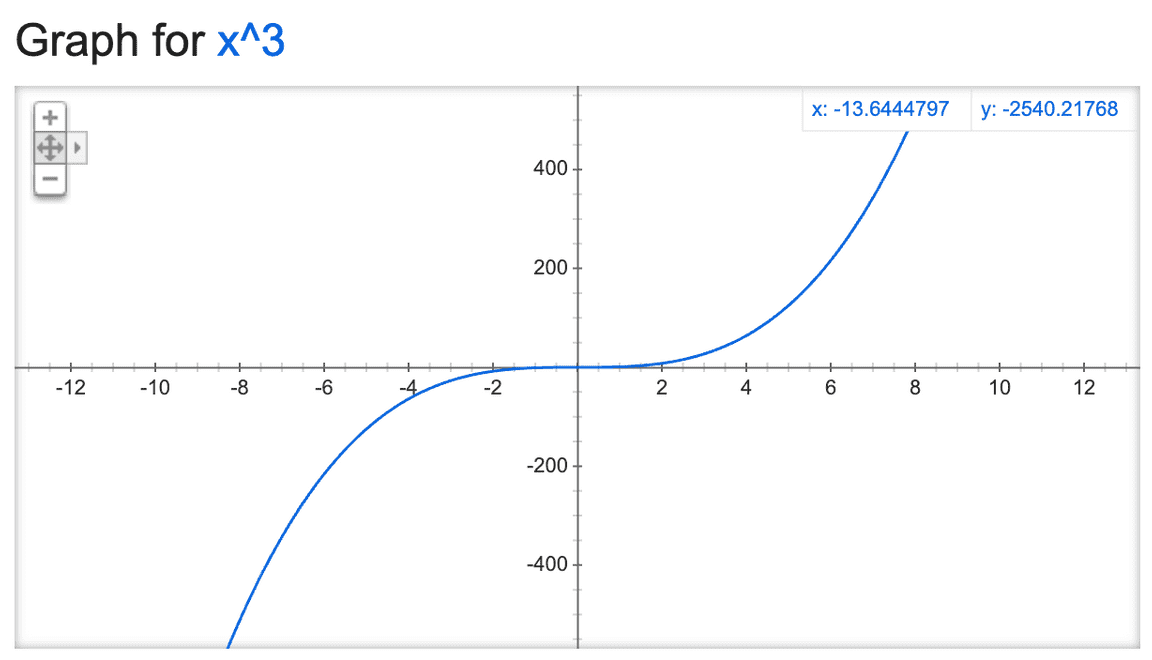## Parabolic Curve Animation With RxJS

rxjsanimationPhoto by SpaceX on Unsplash

Imagine we take a perspective from a slow-motion camera. What humans see as a smooth animation is just an object that is put at different places at every fragment of a time period. This can be expressed as a ‘stream’ of object position. The mechanism of an animation can be simplified by somehow mapping every fragment of a time period to a position point in space. In practice, time cannot be fragmented indefinitely, what we want is an approximation of an “atomic time unit”. The browser has provided us a tool to achieve this, which is the `requestAnimationFrame` API.

We can map every timestamp emitted by `requestAnimationFrame` to a position coordinate at the screen. Let’s see how we can do this in Rxjs!

## Generating time sequence

`.css-137v5jh{margin:-0.8rem 0 0 -0.8rem;}.css-110jbbp{box-sizing:border-box;margin:0;min-width:0;margin-bottom:0.8rem;margin:-0.8rem 0 0 -0.8rem;}.css-1sisz9d{padding:0.4rem;border-radius:0 0 0.8rem 0;vertical-align:top;color:black;background-color:#f1e05a;}.css-187p66r{box-sizing:border-box;margin:0;min-width:0;font-size:1.2rem;padding:0.4rem;border-radius:0 0 0.8rem 0;vertical-align:top;color:black;background-color:#f1e05a;}JavaScript// I only demonstrate the import part once,// they will be omitted in later code.import {  interval,  animationFrameScheduler,  fromEvent,  defer,  merge,} from 'rxjs';import { map, takeWhile, tap, flatMap } from 'rxjs/operators';function duration(ms) {  return defer(() => {    const start = Date.now();    return interval(0, animationFrameScheduler).pipe(      map(() => (Date.now() - start) / ms),      takeWhile(n => n <= 1)    );  });}`

What `defer` does is to only create a new observable upon being subscribed. This is to ensure every subscriber gets a new observable, otherwise, we’ll see weird movements.

First, we record the animation beginning time, then we return an interval function that will emit an event every 0 seconds. This seems ridiculous. However, notice the `animationFrameScheduler`, it schedules at which point the `interval` function can emit an event. This is how we simulate an atomic time unit. The `map` function maps every time unit emitted by `interval` to a time ratio of current elapse to the whole animation duration. `takeWhile` ensures we unsubscribe to `interval` once we reach the end. We know we’ve reached the end if the current elapse equals the total time.

Then we calculate how far the object is away from the origin at every point in time.

## Move the object

`JavaScriptconst distance = d => t => d * t;`

`d` is the total distance the object is going to move. `t` is the time ratio, which has been calculated in the last step. We multiply them and get the distance at that point.

We get the target in the DOM and move it.

`JavaScriptconst targetDiv = document.querySelector('.target');const moveRight\$ = duration(1500).pipe(  map(distance(1000)),  tap(x => (targetDiv.style.left = x + 'px')));const moveDown\$ = duration(900).pipe(  map(distance(700)),  tap(y => (targetDiv.style.top = y + 'px')));`

The first stream moves the object to the right, the second to the bottom. Notice that the animation hasn’t taken place, because we haven’t’ subscribed to them yet.

We combine these two streams into a new stream, making the object move rightwards and downwards at the same time.

`JavaScriptmerge(moveRight\$, moveDown\$).subscribe();`

This is boring, we don’t see any curve yet. But bear with me.

## Make the motion trajectory parabolic!

I hope you still remember middle school math. If you don’t, fret not. It’s pretty intuitive actually. What we observe as a curve movement is the result of an object moves at different speeds in different directions. The shape of the curve can be expressed in a mathmatical equation. Take a look of the graph of the equation y = x^3, the left and right half of it is the parabolic curve we want:If we can make the downward speed and rightward speed form a cubic equation, then we have a parabolic curve movement!

We can use two different easing functions that form a cubic relationship between them:

The first one is `easeInQuad`

`JavaScriptconst easeInQuad = t => t * t;`

The second one is `easeInQuint`

`JavaScriptconst easeInQuint = t => Math.pow(t, 6);`

Then we only need to map the time ratio emitted from the interval pipeline to the result of applying these easing functions:

`JavaScriptconst moveDown\$ = duration(900).pipe(  map(easeInQuint),  map(distance(700)),  tap(y => (targetDiv.style.top = y + 'px')));const moveRight\$ = duration(1500).pipe(  map(easeInQuad),  map(distance(1000)),  tap(x => (targetDiv.style.left = x + 'px')));`

See the codepen below for the result and the complete code:

How to Write Recursion Like A ProIntroduction to Lenses in JavaScript
Back to Home
Design and </> with ☕ by Lei Huang